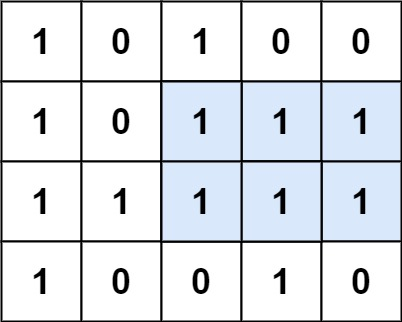| English | 简体中文 |

# 85. Maximal Rectangle

## Description

Given a rows x cols binary matrix filled with 0's and 1's, find the largest rectangle containing only 1's and return its area.

Example 1:Input: matrix = [["1","0","1","0","0"],["1","0","1","1","1"],["1","1","1","1","1"],["1","0","0","1","0"]]
Output: 6
Explanation: The maximal rectangle is shown in the above picture.


Example 2:

Input: matrix = [["0"]]
Output: 0


Example 3:

Input: matrix = [["1"]]
Output: 1


Constraints:

• rows == matrix.length
• cols == matrix[i].length
• 1 <= row, cols <= 200
• matrix[i][j] is '0' or '1'.